Tamil Nadu Board of Secondary EducationSSLC (English Medium) Class 8

# Central Angle and the Measure of an Arc

## Definition

Central Angle: An angle whose vertex is the centre of the circle is called a central angle.

## Notes

### Central Angle and the Measure of an Arc: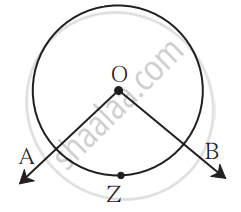An angle whose vertex is the centre of the circle is called a central angle.
In the figure, ‘O’ is the vertex of the ∠AOB.

The ∠AOB in the figure is the central angle corresponding to arc AZB. The measure of the angle subtended at the centre by an arc is taken to be the measure of the arc.

#### 1. The measure of a minor arc: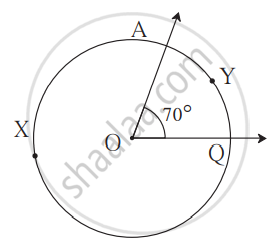In the above figure, the measure of ∠AOQ = 70°.
∴ Measure of the minor arc AYQ is 70°.
It is written as m(arc AYQ) = 70°.

#### 2. The measure of a major arc:

Measure of a major arc = 360° - measure of the corresponding minor arc
∴ Measure of major arc AXQ in the figure = 360° - 70° = 290°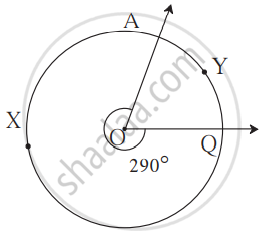#### 3. The measure of a circle: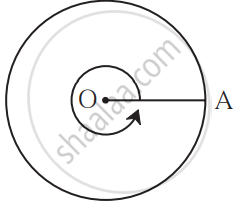When the radius OA of a circle turns anti-clockwise, as shown in the above figure, through a complete angle, it turns through an angle that measures 360°. Its endpoint A completes one circle. The angle subtended at the center by the circle is 360°.
∴ The measure of the complete circle is 360°.

#### 4. Measure of a semicircular arc: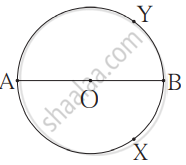The measure of a semicircular arc = 180°.

If you would like to contribute notes or other learning material, please submit them using the button below.

### Shaalaa.com

Central Angles, Circle Arcs, Angle Measurement, Major Arcs vs Minor Arcs [00:13:45]
S
0%